Lognormal Distribution

#Lognormal Distribution

The Lognormal Distribution represents a Normal Distribution on the natural log of a random variable. It is very common and used in a wide variety of industries to model data which is skewed either positively or negatively. In meteorology it is used to model monthly or yearly rainfall. In the oil and gas industry it is used to model the level of reserves. In the health care industry it is used to represent the duration of sickness absence and physicians' consultation time. In economic theory, the distribution of income in developed countries is often said to be lognormal. The Lognormal Distribution is also commonly employed in engineering for topics ranging from fatigue failures and yield stress to dye-dillution curves. In failure analysis the Lognormal Distribution is sometimes seen as a competitor to the Weibull Distribution for representing lifetime distributions of manufactured products.

The Lognormal Distribution can be derived from the normal distribution. It has two parameters, mu and sigma, similar to the parameters of a normal distribution. The parameter mu can have any value, but sigma must be positive. The lognormal distribution is: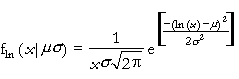where x>0 and sigma>0.

The approximation procedure in DPL will not work for a lognormal distribution if its parameters are too large. In particular, the value of sigma, which must be positive, cannot be larger than about 5. The other parameter, mu, can be positive or negative, but its absolute value cannot exceed a fairly small number. That number depends on the value of sigma, but a practical limit is around 100. In practice, you should never have parameters as large as these limits.

The equations for the mean and variance are: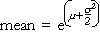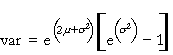The equations for the parameters are: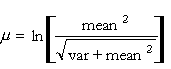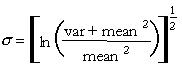For most realistic values of the mean and variance, these equations produce small values of mu and sigma. In particular, sigma is usually less than 3.

Versions: DPL Professional, DPL Enterprise, DPL Portfolio